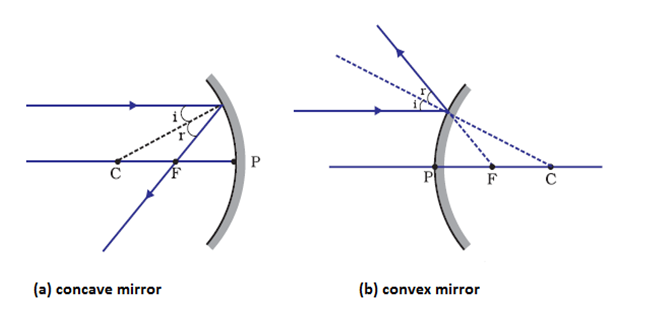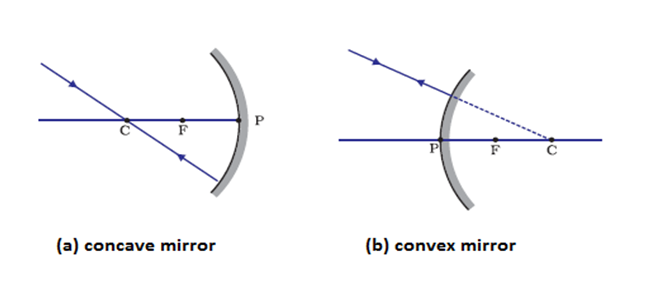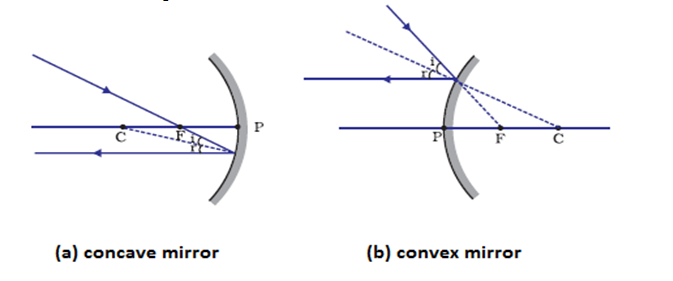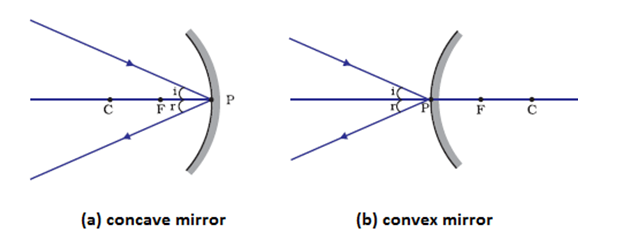# Reflection of light Notes for Class 10

## Image Formation by Spherical mirrors

• The nature, position and size of the image formed by a concave mirror depend on the position of the object in relation to points P, F and C.
• The image formed can be real as well as virtual depending on the positions of the object.
• The image is either magnified, reduced or has the same size, depending on the position of the object.

## Rules for obtaining images formed by spherical mirrors

#### (1) Rule 1

A ray of light which is parallel to the principle axis of the mirror passes through its focus after reflection from the mirror as shown below in the figureFrom the figure given above it can be clearly seen that the light rays passes through principle focus in case of concave mirrors and appears to diverge from principle focus in case of concave mirror.

#### (2) Rule 2

A ray of light passing through the centre of curvature of the curvature of the concave mirror or directed in the direction of the centre of curvature of a convex mirror, is reflected back along the same path as shown below in the figureThis happens because the incident rays fall on the mirror along the normal to the reflecting surface.

#### (3) Rule 3

A ray passing through principle focus of a concave mirror or a ray which is directed towards the principal focus of a convex mirror, becomes parallel to the principle axis after reflection and is shown below in the figure#### (4) Rule 4

A ray incident obliquely to the principal axis, towards a point P (pole of the mirror), on the concave mirror or a convex mirror, is reflected obliquely. The incident and reflected rays follow the laws of reflection at the point of incidence (point P), making equal angles with the principal axis and is shown below in the figureGiven below are the links of some of the reference books for class 10 Science.

You can use above books for extra knowledge and practicing different questions.

### Practice Question

Question 1 Which among the following is not a base?
A) NaOH
B) $NH_4OH$
C) $C_2H_5OH$
D) KOH
Question 2 What is the minimum resistance which can be made using five resistors each of 1/2 Ohm?
A) 1/10 Ohm
B) 1/25 ohm
C) 10 ohm
D) 2 ohm
Question 3 Which of the following statement is incorrect? ?
A) For every hormone there is a gene
B) For production of every enzyme there is a gene
C) For every molecule of fat there is a gene
D) For every protein there is a gene

Note to our visitors :-

Thanks for visiting our website.